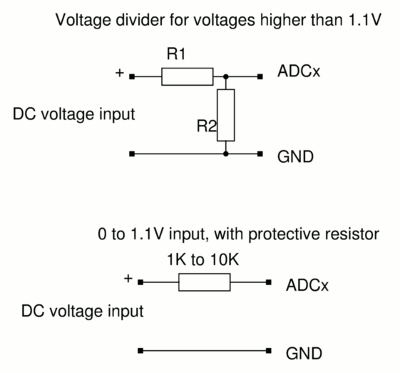# Voltage divider calculator for the max-adc boardThe analog inputs on the ethernet board accept voltages between 0V and 1.1V with a digital reading of 2046 corresponding to 1.1V input. The extended precision from 0 to 2046 is achieved trough oversampling. Theoretically you can connect any power source that provices less than 1.1V directly however if the voltage is ever accidently much higher than 1.1V then one can easily damage the hardware. It is therefore a good idea to add a 1K to 10K resistor in series. This resistor will not influence the voltage reading and just protect the board.

To measure higher DC voltages a voltage divider circuit considting of two resistors R1 and R2 can be added.

## Voltage divider calculator

Enter the values for R1 and R2 and the calculator will calculate the maximum input voltage.
Formula: Uin=1.1V * (R1+R2) / R2

 R1: KOhm [suggested range: 100-500] R2: KOhm [suggested range: 10-500] Resulting input voltage range 0V to: V